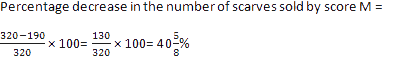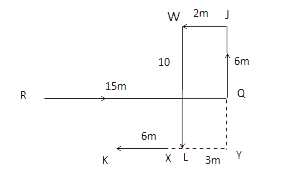# Nucleus-Software Reasoning Question

DIRECTIONS for question 1 to 4:Refer to the graph and answer the given question:1. What is the difference between the total number of scarves sold by store M in 2003 and 2004 together and total number of scarves sold by store N in 2005 and 2006 together?
1. 160
2.  100
3.  140
4.  150
5.  120
Total Number of scarves sold store M in 2003 and 2004= 190+320= 510
Total Number of scarves sold store M in 2005 and 2006=260+370= 630
Difference between the total Number of scarves sold store M in 2003 & 2004 and Total Number of scarves sold store N in 2005 and 2006 is = 630 – 510 = 120.
2. Number of scarves sold by store M decreased by what percent from 2004 to 2005?
1. 40 5/8
2.  45 3/8
3. 42 3/8
4.  30 3/8
5.  35 5/81.  430
2. 450
3.  420
4.  460
5. 440
Ratio between total number scarves sold by stores M & N together in 2002 and that in 2009 is 15:11.
Total number scarves sold by stores M & N together in 2002 = 240+360 = 600
According to question, 600/X= 15/11
Or, x = 440
Total number scarves sold by stores M & N together in 2009 = 440.
• If the total number of scarves sold by stores M and N together in 2008 is 10% more than that in 2006, what is the total number of scarves sold by stores M and N together in 2008?
1. 638
2. 406
3. 414
4. 396
5.  408
Total number of scarves sold by stores M & N in 2006 = 210+370 = 580
Total number of scarves sold by stores M & N in 2008 = 580×110/100 = 638
DIRECTIONS for question 5 to 7:Study the given information carefully to answer the given question.
R is 15m west of Q. J is 6m north of Q. W is 2m west of J. L is 10m south of W. K is 6m west of L.
• If F is 4m to the south of R and V is 2m east of K, how far is Point F from Point V?
1. 8m
2. 11m
3. 5m
4.  9m
5.  4m
The following diagram can be formed with the given information.
Now, FL = RQ – WJ = 15 – 2 = 13mFurther, FV = FL – VL = 13 – 4 = 9m

• Kabir walks 10m towards south from Point J, takes a right turn and walks for 3m. How far will he be from Point K?
1.  4m
2. 10m
3.  9m
4.  6m
5. 5m
As shown in the diagram, if Kabir walks 10 m towards south from point J, he will reach at point Y. Then, moving 3 m to the right side, he will finally reach point X.
Now, XL = XY – LY = 3 – 2 = 1 m
And distance between X and K = KL – XL = 6 – 1 = 5m• In which direction is R with respect to J?
1. West
2.  South east
3. North east
4. North
5. South west
As per the diagram, it can be observed that R is towards South-west with respect to J.DIRECTIONS for question 8 to 10:What will come in place of question mark (?) in the given number series?
• 700  457  376  349  340  ?
1. 266
2. 329
3. 304
4. 337
5. 307
Given series is:
700                  457                          376                          349                     340                  ?
Pattern is 700 – 457 = 243, 457 – 376 = 81, 376 – 349 = 27, 349 – 340 = 9
Thus, the next term is 340 – 3 = 337
• 1  2  6  21  88  ?
1.  425
2.  475
3. 295
4.  445
5.  395
Given series is:
1             2            6           21          88           ?
Pattern is 1×1+1= 2
2×2+2= 6
6×3+3=21
21×4+4= 88
88×5+5= 445
So the next term of the series is = 445
• 19  20  16  25  9  ?
1. 45
2. 55
3. 59
4.  34
5.  81